# Test: Graphs- 2

## 20 Questions MCQ Test GATE Computer Science Engineering(CSE) 2023 Mock Test Series | Test: Graphs- 2

Description
Attempt Test: Graphs- 2 | 20 questions in 60 minutes | Mock test for Computer Science Engineering (CSE) preparation | Free important questions MCQ to study GATE Computer Science Engineering(CSE) 2023 Mock Test Series for Computer Science Engineering (CSE) Exam | Download free PDF with solutions
QUESTION: 1

Solution:
QUESTION: 2

Solution:
QUESTION: 3

### What are the appropriate data structures for following algorithms? 1) Breadth First Search 2) Depth First Search 3) Prim's Minimum Spanning Tree 4) Kruskal' Minimum Spanning Tree

Solution:

1) Breadth First Search uses Queue
2) Depth First Search uses Stack
3) Prim's Minimum Spanning Tree uses Priority Queue.
4) Kruskal' Minimum Spanning Tree uses Union Find.

QUESTION: 4

The Breadth First Search algorithm has been implemented using the queue data structure. One possible order of visiting the nodes of the following graph is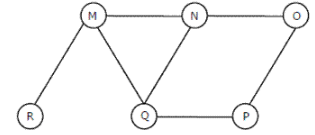Solution:

Option (A) is MNOPQR. It cannot be a BFS as the traversal starts with M, but O is visited before N and Q. In BFS all adjacent must be visited before adjacent of adjacent. Option (B) is NQMPOR. It also cannot be BFS, because here, P is visited before O. (C) and (D) match up to QMNP. We see that M was added to the queue before N and P (because M comes before NP in QMNP). Because R is M's neighbor, it gets added to the queue before the neighbor of N and P (which is O). Thus, R is visited before O.

QUESTION: 5

Let G be an undirected graph. Consider a depth-first traversal of G, and let T be the resulting depth-first search tree. Let u be a vertex in G and let v be the first new (unvisited) vertex visited after visiting u in the traversal. Which of the following statements is always true? (GATE CS 2000)

Solution:

In DFS, if 'v' is visited after 'u', then one of the following is true.

1) (u, v) is an edge.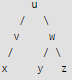2) 'u' is a leaf node.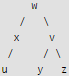In DFS, after visiting a node, we first recur for all unvisited children. If there are no unvisited children (u is leaf), then control goes back to parent and parent then visits next unvisited children.

QUESTION: 6

Consider the following graph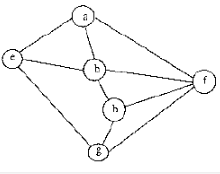Among the following sequences
I) a b e g h f
II) a b f e h g
III) a b f h g e
IV) a f g h b e

Q. Which are depth first traversals of the above graph?

Solution:

We can check all DFSs for following properties.

In DFS, if a vertex 'v' is visited after 'u', then one of the following is true.

1) (u, v) is an edge.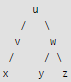2) 'u' is a leaf node.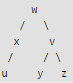In DFS, after visiting a node, we first recur for all unvisited children. If there are no unvisited children (u is leaf), then control goes back to parent and parent then visits next unvisited children.

QUESTION: 7

Make is a utility that automatically builds executable programs and libraries from source code by reading files called makefiles which specify how to derive the target program. Which of the following standard graph algorithms is used by Make.

Solution:

Make can decide the order of building a software using topological sorting. Topological sorting produces the order considering all dependencies provide by makefile. See following for details. Topological Sorting

QUESTION: 8

Given two vertices in a graph s and t, which of the two traversals (BFS and DFS) can be used to find if there is path from s to t?

Solution:

We can use both traversals to find if there is a path from s to t.

QUESTION: 9

Which of the following condition is sufficient to detect cycle in a directed graph?

Solution:
QUESTION: 10

Is following statement true/false If a DFS of a directed graph contains a back edge, any other DFS of the same graph will also contain at least one back edge

Solution:

A back edge means a cycle in graph. So if there is a cycle, all DFS traversals would contain at least one back edge.

QUESTION: 11

Is following statement true/false? A DFS of a directed graph always produces the same number of tree edges, i.e., independent of the order in which vertices are considered for DFS.

Solution:

Consider the following graph. If we start from 'a', then there is one tree edge. If we start from 'b', then there is no tree edge. a---->b

QUESTION: 12

If the DFS finishing time f[u] > f[v] for two vertices u and v in a directed graph G, and u and v are in the same DFS tree in the DFS forest, then u is an ancestor of v in the depth first tree.

Solution:

In a graph with three nodes, r u and v, with edges (r; u) and (r; v), and r is the starting point for the DFS, u and v are siblings in the DFS tree, neither as the ancestor of the other.

QUESTION: 13

Consider the DAG with Consider V = {1, 2, 3, 4, 5, 6}, shown below. Which of the following is NOT a topological ordering?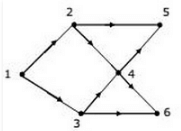Solution:

In option D, 1 appears after 2 and 3 which is not possible in Topological Sorting. In the given DAG it is directly visible that there is an outgoing edge from vertex 1 to vertex 2 and 3 hence 2 and 3 cannot come before vertex 1 so clearly option D is incorrect topological sort. But for questions in which it is not directly visible we should know how to find topological sort of a DAG.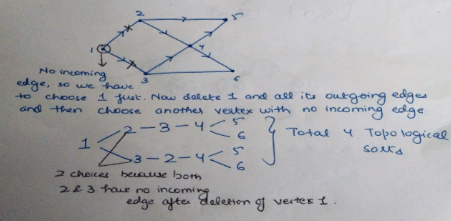QUESTION: 14

Let G be a graph with n vertices and m edges. What is the tightest upper bound on the running time on Depth First Search of G? Assume that the graph is represented using adjacency matrix.

Solution:

Depth First Search of a graph takes O(m+n) time when the graph is represented using adjacency list. In adjacency matrix representation, graph is represented as an "n x n" matrix. To do DFS, for every vertex, we traverse the row corresponding to that vertex to find all adjacent vertices (In adjacency list representation we traverse only the adjacent vertices of the vertex). Therefore time complexity becomes O(n2)

QUESTION: 15

Consider the tree arcs of a BFS traversal from a source node W in an unweighted, connected, undirected graph. The tree T formed by the tree arcs is a data structure for computing.

Solution:

BFS always produces shortest path from source to all other vertices in an unweighted graph.

QUESTION: 16

Suppose depth first search is executed on the graph below starting at some unknown vertex. Assume that a recursive call to visit a vertex is made only after first checking that the vertex has not been visited earlier. Then the maximum possible recursion depth (including the initial call) is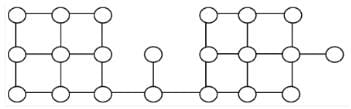Solution:

The following diagram shows the worst case situation where the recursion tree has maximum depth.So the recursion depth is 19 (including the first node).

QUESTION: 17

Let T be a depth first search tree in an undirected graph G. Vertices u and n are leaves of this tree T. The degrees of both u and n in G are at least 2. which one of the following statements is true?

Solution:

Below example shows that A and B are FALSE: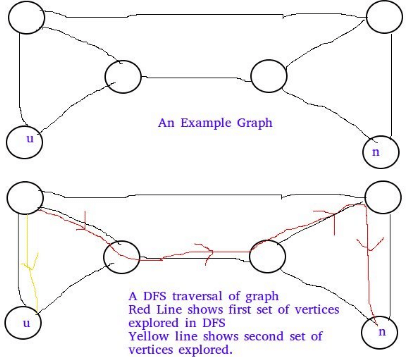Below example shows C is false: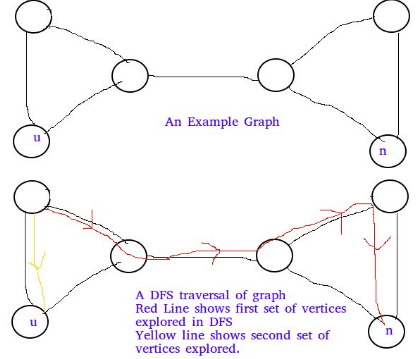QUESTION: 18

Let G be an undirected graph. Consider a depth-first traversal of G, and let T be the resulting depth-first search tree. Let u be a vertex in G and let v be the first new (unvisited) vertex visited after visiting u in the traversal. Which of the following statements is always true?

Solution:

In DFS, if 'v' is visited after 'u', then one of the following is true.
1) (u, v) is an edge.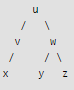2) 'u' is a leaf node.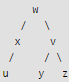In DFS, after visiting a node, we first recur for all unvisited children. If there are no unvisited children (u is leaf), then control goes back to parent and parent then visits next unvisited children.

QUESTION: 19

In a depth-first traversal of a graph G with n vertices, k edges are marked as tree edges. The number of connected components in G is

Solution:

Tree edges are the edges that are part of DFS tree.  If there are x tree edges in a tree, then  x+1 vertices in the tree. The output of DFS is a forest if the graph is disconnected.  Let us see below simple example where graph is disconnected.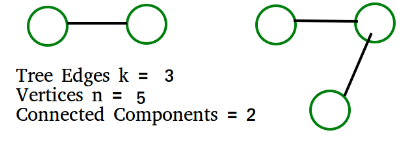The above example matches with D option More Examples: 1) All vertices  of Graph are connected.  k must be n-1.  We get number of connected components  = n- k =  n - (n-1) = 1 2) No vertex is connected. k must be 0.  We get number of connected components  = n- k =  n - 0 = n

QUESTION: 20

Consider the following directed graph.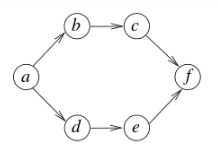Q. The number of different topological orderings of the vertices of the graph isUse Code STAYHOME200 and get INR 200 additional OFF Use Coupon Code